Courses
Courses for Kids
Free study material
Offline Centres
More

# Geometric Solid – Meaning, Definition, and Solved ExamplesLast updated date: 30th Nov 2023
Total views: 153.6k
Views today: 2.53k## What Does Geometry Mean?

We call geometry a branch of Math that focuses on the measurement and relationship of lines, angles, surfaces, solids, and points. An example of geometry is the calculation of a triangle's angles. Shapes that we study in geometry are 2-d and 3-d.

2-d shapes have two dimensions, such as x and the y-axis. Also, it has length and width. Examples are circle, square, rectangle, and so on. However, shapes like sphere, hemisphere, cylinder, cube, and cuboid have three dimensions, i.e., x, y, and z-axis. The walls of your room have a 3-d structure. Also, these shapes have length, height or depth, and width.

The walls of your room have a kind of 3-d space which you live in. And the geometry of this 3-d space is called the solid geometry or simply we can say it is a geometric solid.

## What is Geometric Solid?

Before starting with what solid geometry is, let us go through some of the common 3-d shapes:Image: Solid common 3-d shapes

We understood from the above text that these shapes have three dimensions having length, depth, and width. These shapes carry the following properties:

• Capacity or volume (think of how much water it could hold).

• Surface area (think of the amount of area covered by the surface of something).

• How many vertices (corner points), faces, and edges do they have.

## Formulas of Solid Geometry

The following table gives the volume formulas and surface area formulas for the following 3-D solid shapes:

 3-D shape Image Surface Area Formula Volume Formula Cube with side ‘s’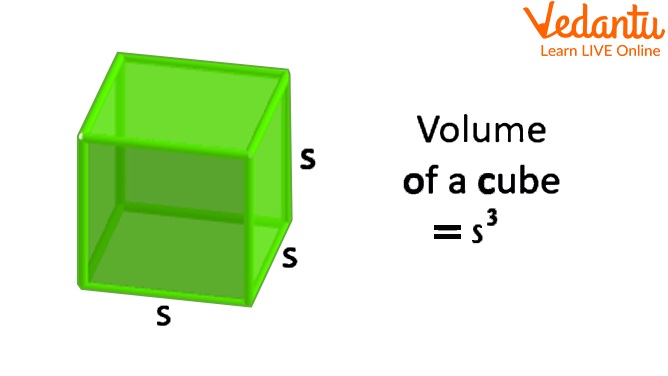6s2 s3 Rectangular prism with length l, height h, and width w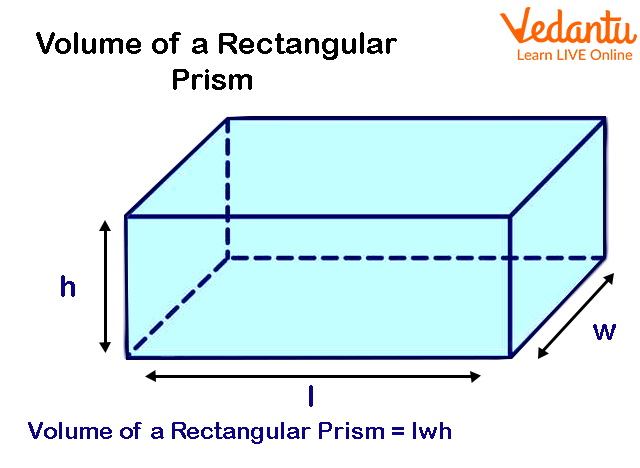2 (lw + wh + hl) lwh Prismwith an area of base ‘B’, the perimeter of base B, ‘P’, and height ‘h’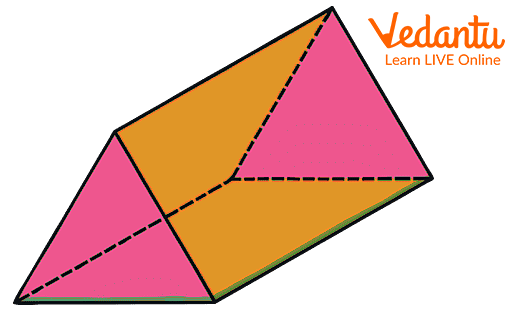2B + PH Bh Cylinderradius = r, height = h2πr2 + 2πrh πr2h Sphereradius = r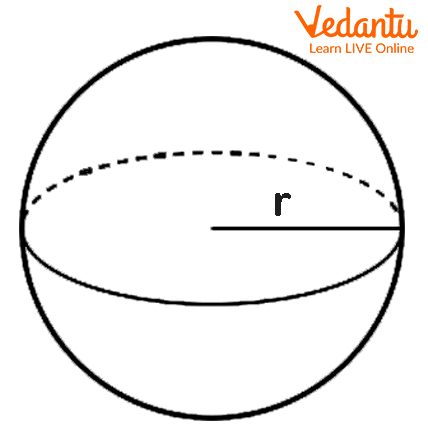4πr2 4/3 πr3 Coneradius = r, vertical height = h, slant height = h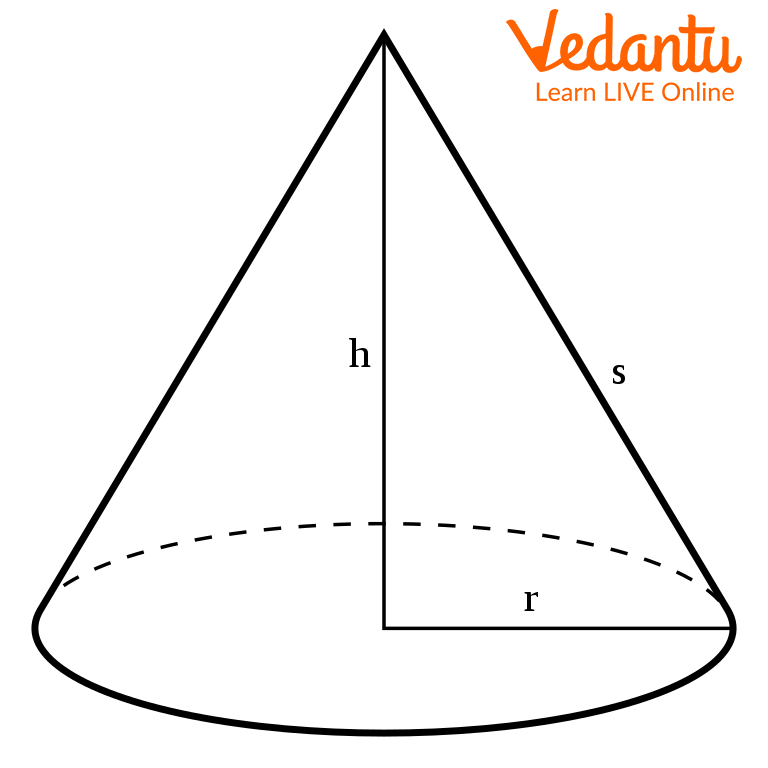πr2 + πrs ⅓ πr2h Pyramidwith an area of base B, the perimeter of base P, vertical height h, and slant height s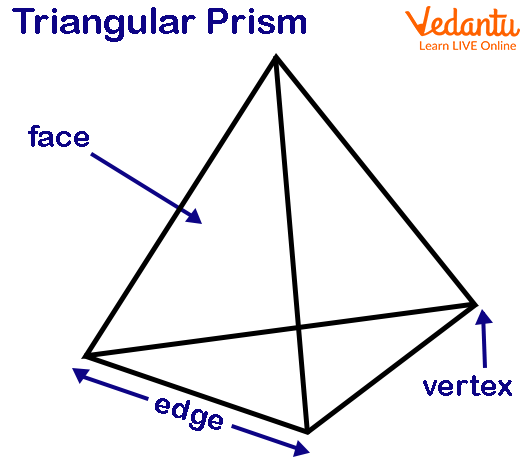Regular pyramidB + 1 p/s ⅓ bh

## Solved Examples of Geometric Solid

1. Find the volume and surface area of a cube whose side is 7 cm.

Solution:

Side, a = 7 cm.

The volume of a cube = a3 cubic units

V = 73

V = 7 x 7 x 7

V = 343 cm3

Therefore, the volume of a cube is 343 cubic centimeter.

The surface area of a cube = 6a2 square units

SA = 6 x (7)2 cm2

SA = 6 x (49)

SA = 294 cm2

Therefore, the surface area of a cube is 294 square centimeters.

2. Find the total surface area of a cuboid of dimensions 9 cm × 8 cm × 6 cm.

Solution:

Given dimensions of a cuboid: 9 cm x 8 cm x 6 cm

That means length = l = 9 cm, breadth = b = 8 cm, and height = h = 6 cm.

Total surface area of a cuboid = 2 (lb + bh +hl)

= 2 (9 x 8 + 8 x 6 + 6 x 9)

= 2 (72 + 48 + 54 )

= 348 cm2

Hence, the total surface area of the cuboid is 348 cm2.

From the above text on geometric solid, we understand that solid geometry is regarded with 3-D shapes. Examples of three-dimensional solid shapes are cubes, rectangular solids, prisms, cylinders, spheres, cones, and pyramids.

## FAQs on Geometric Solid – Meaning, Definition, and Solved Examples

1. What are the properties of geometric shapes?

Below is the list of properties geometric shapes hold:

• Cube: face – 6 squared faces, vertices – 8, edges – 12.

• Cuboid: face – 6 rectangular faces, vertices – 8, edges – 12.

• Sphere: curved surface – 1, edges – 0, vertices – 0.

• Cylinder: flat surface – 2, curved surface – 1, face – 3, edges – 2, vertices – 0.

• Cone: flat surface – 1, curved surface – 1, face – 2, edges – 1, vertices – 1.

2. How do we identify geometric shapes?

Geometric shapes are Mathematical shapes that are perfect and regular. These shapes are characterized by straight lines, angles, and points. Examples are squares, rectangles, triangles, parallelograms, hexagons, etc.

However, an exception to geometric shapes would be a perfect circle as it has no straight lines or points.